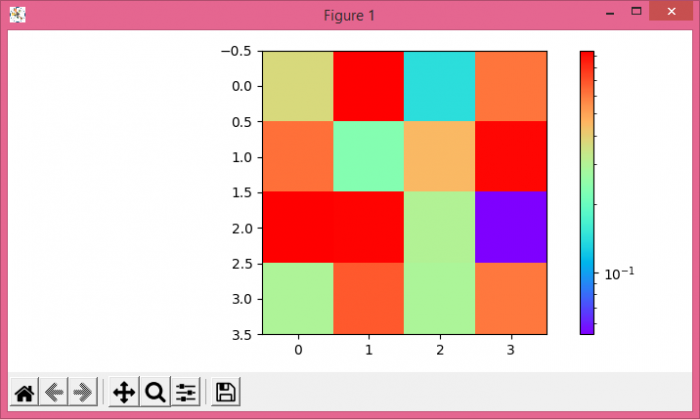# How to draw a log-normalized imshow plot with a colorbar representing the raw data in Matplotlib?

To draw a log-normalized imshow() plot with a colorbar representing the raw data in matplotlib, we can take the following steps −

• Create a 2D array using numpy.
• Display the data as an image, i.e., on a 2D regular raster, using imshow() method
• Create a colorbar for a ScalarMappable instance, *mappable*, using imshow() method.
• To display the figure, use show() method.

## Example

import numpy as np
from matplotlib import pyplot as plt, cm
from matplotlib import colors
plt.rcParams["figure.figsize"] = [7.00, 3.50]
plt.rcParams["figure.autolayout"] = True
data = np.random.rand(4, 4)
im = plt.imshow(data, cmap=cm.rainbow, norm=colors.LogNorm())
plt.colorbar(im)
plt.show()

## Output